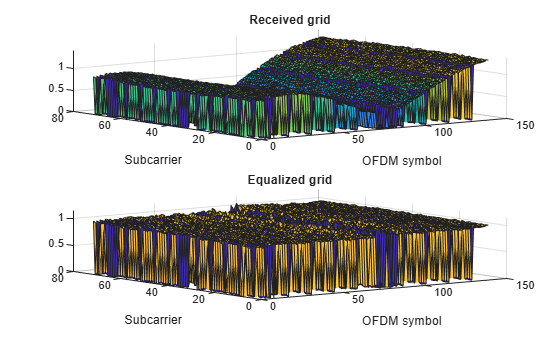# lteEqualizeMMSE

MMSE equalization

## Syntax

``````[out,csi] = lteEqualizeMMSE(rxgrid,channelest,noiseest)``````

## Description

example

``````[out,csi] = lteEqualizeMMSE(rxgrid,channelest,noiseest)``` returns equalized data in multidimensional array, `out`. MMSE equalization is applied to the received data resource grid in the matrix, `rxgrid`, using the channel information in the `channelest` matrix. `noiseest` is an estimate of the received noise power spectral density. Alternatively, the input `channelest` can be provided as a 3-D array of size NRE-by-`NRxAnts`-by-P, and the input `rxgrid` can be provided as a matrix of size NRE-by-`NRxAnts`. In this case, the first two dimensions have been reduced to one dimension by appropriate indexing through the frequency and time locations of the resource elements of interest, typically for a single physical channel. The outputs, `out` and `csi`, are of size (N×M)-by-P. ```

## Examples

collapse all

Equalize the received signal for RMC R.4 after channel estimation. Use the MMSE equalizer.

Create cell-wide configuration structure and generate transmit signal. Configure propagation channel.

```enb = lteRMCDL('R.4'); [txSignal,~,info] = lteRMCDLTool(enb,[1;0;0;1]); chcfg.DelayProfile = 'EPA'; chcfg.NRxAnts = 1; chcfg.DopplerFreq = 70; chcfg.MIMOCorrelation = 'Low'; chcfg.SamplingRate = info.SamplingRate; chcfg.Seed = 1; chcfg.InitPhase = 'Random'; chcfg.InitTime = 0; txSignal = [txSignal; zeros(15,1)]; N = length(txSignal); noise = 1e-3*complex(randn(N,chcfg.NRxAnts),randn(N,chcfg.NRxAnts)); rxSignal = lteFadingChannel(chcfg,txSignal)+noise;```

Perform synchronization and OFDM demodulation.

```offset = lteDLFrameOffset(enb,rxSignal); rxGrid = lteOFDMDemodulate(enb,rxSignal(1+offset:end,:));```

Create channel estimation configuration structure and perform channel estimation.

```cec.FreqWindow = 9; cec.TimeWindow = 9; cec.InterpType = 'Cubic'; cec.PilotAverage = 'UserDefined'; cec.InterpWinSize = 3; cec.InterpWindow = 'Causal'; [hest,noiseEst] = lteDLChannelEstimate(enb, cec, rxGrid);```

Equalize and plot received and equalized grids.

```eqGrid = lteEqualizeMMSE(rxGrid, hest, noiseEst); subplot(2,1,1) surf(abs(rxGrid)) title('Received grid') xlabel('OFDM symbol') ylabel('Subcarrier') subplot(2,1,2) surf(abs(eqGrid)) title('Equalized grid') xlabel('OFDM symbol') ylabel('Subcarrier')```This example applies MMSE equalization on the received signal for reference measurement channel (RMC) R.5, after channel estimation.

Set the DL reference measurement channel to R.5

`enb = lteRMCDL('R.5');`

Set channel estimator configuration `PilotAverage` field to `UserDefined`. as follows: averaging window of 9 resource elements in both frequency and time domain, cubic interpolation with a casual window.

```cec = struct('FreqWindow',9,'TimeWindow',9,'InterpType','cubic'); cec.PilotAverage = 'UserDefined'; cec.InterpWinSize = 1; cec.InterpWindow = 'Causal';```

Generate the `txWaveform`.

```txWaveform = lteRMCDLTool(enb,[1;0;0;1]); n = length(txWaveform);```

Apply some random noise to the transmitted signal and save as the `rxWaveform`.

`rxWaveform = repmat(txWaveform,1,2)+complex(randn(n,2),randn(n,2))*1e-3;`

`rxGrid = lteOFDMDemodulate(enb,rxWaveform);`

Then, perform channel estimation.

`[hest,n0] = lteDLChannelEstimate(enb,cec,rxGrid);`

Finally, apply the MMSE equalization.

`out = lteEqualizeMMSE(rxGrid,hest,n0);`

Show scatter plot of one component carrier.

`scatterplot(out(:,1))`## Input Arguments

collapse all

Received data resource grid, specified as a 3-D numeric array or a 2-D numeric matrix. As a 3-D numeric array, it has size N-by-M-by-`NRxAnts`, where N is the number of subcarriers, M is the number of OFDM symbols, and `NRxAnts` is the number of receive antennas.

Alternatively, as a 2-D numeric matrix, it has size NRE-by-`NRxAnts`. In this case, the first two dimensions have been reduced to one dimension by appropriate indexing through the frequency and time locations of the resource elements of interest, typically for a single physical channel.

Data Types: `double`
Complex Number Support: Yes

Channel information, specified as a 4-D numeric array or a 3-D numeric array. As a 4-D numeric array, it has size N-by-M-by-`NRxAnts`-by-P. N is the number of subcarriers, M is the number of OFDM symbols, `NRxAnts` is the number of receive antennas, and P is the number of transmit antennas. Each element is a complex number representing the narrowband channel for each resource element and for each link between transmit and receive antennas. This matrix can be obtained using the channel estimation command `lteDLChannelEstimate`.

Alternatively, as a 3-D numeric array, it has size NRE-by-`NRxAnts`-by-P. In this case, the first two dimensions have been reduced to one dimension by appropriate indexing through the frequency and time locations of the resource elements of interest, typically for a single physical channel.

Data Types: `double`
Complex Number Support: Yes

Noise power estimate, specified as a numeric scalar. It is an estimate of the received noise power spectral density per RE on `rxgrid`.

Data Types: `double`

## Output Arguments

collapse all

Equalized output data, returned as a 3-D numeric array or a 2-D numeric matrix. As a 3-D numeric array, it has size N-by-M-by-P, where N is the number of subcarriers, M is the number of OFDM symbols, and P is the number of transmit antennas.

Alternatively, if `channelest` is provided as a 3-D array, `out` is a 2-D numeric matrix of size (N×M)-by-P. In this case, the first two dimensions have been reduced to one dimension by appropriate indexing through the frequency and time locations of the resource elements of interest, typically for a single physical channel.

Data Types: `double`
Complex Number Support: Yes

Soft channel state information, returned as a 3-D numeric array of the same size as `out`. As a 3-D numeric array, it has size N-by-M-by-P, where N is the number of subcarriers, M is the number of OFDM symbols, and P is the number of transmit antennas. `csi` provides an estimate (via MMSE) of the received RE gain for each received RE.

Alternatively, if `channelest` is provided as a 3-D array, `csi` is a 2-D numeric matrix of size (N×M)-by-P. In this case, the first two dimensions have been reduced to one dimension by appropriate indexing through the frequency and time locations of the resource elements of interest, typically for a single physical channel.

Data Types: `double`

## Version History

Introduced in R2014a